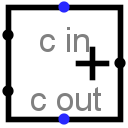#Addierer

 Bibliothek: Arithmetic Eingeführt: 2.0 Beta 11 Aussehen:## Verhalten

This component adds two values coming in via the west inputs and outputs the sum on the east output. The component is designed so that it can be cascaded with other adders to provide add more bits than is possible with a single adder: The carry-in input provides a one-bit value to be added into the sum also (if it is specified), and a carry-out output provides a one-bit overflow value that can be fed to another adder.

If either of the addends contains some floating bits or some error bits, then the component will perform a partial addition. That is, it will compute as many low-order bits as possible. But above the floating or error bit, the result will have floating or error bits.

## Pins

West edge, north end (input, bit width matches Data Bits attribute)
One of the two values to add.
West edge, south end (input, bit width matches Data Bits attribute)
The other of the two values to add.
North edge, labeled c in (input, bit width 1)
A carry value to add into the sum. If the value is unknown (i.e., floating), then it is assumed to be 0.
East edge (output, bit width matches Data Bits attribute)
The lower dataBits bits of the sum of the two values coming in the west edge, plus the cin bit.
South edge, labeled c out (output, bit width 1)
The carry bit computed for the sum. If the values added together as unsigned values yield a result that fits into dataBits bits, then this bit will be 0; otherwise, it will be 1.

## Attribute

When the component is selected or being added, Alt-0 through Alt-9 alter its Data Bits attribute.

Data Bits
The bit width of the values to be added and of the result.

Keines.

## Verhalten des Textwerkzeugs

Keines.

Zurück zur Bibliotheksreferenz